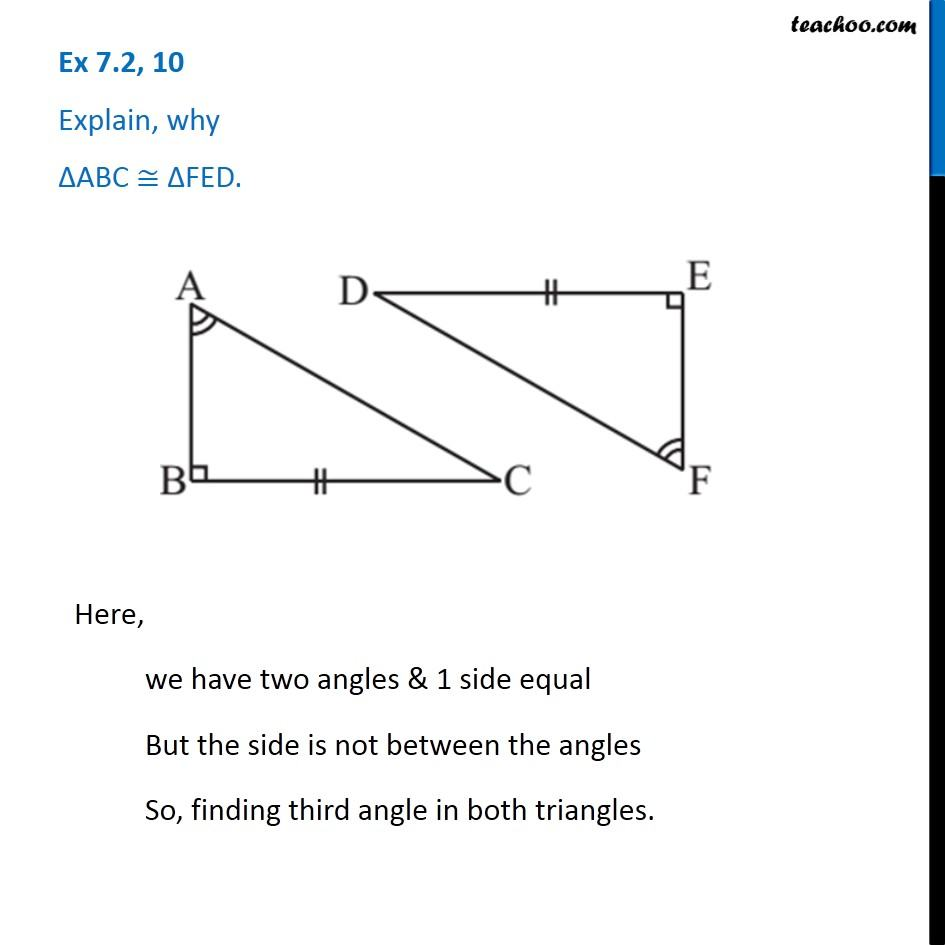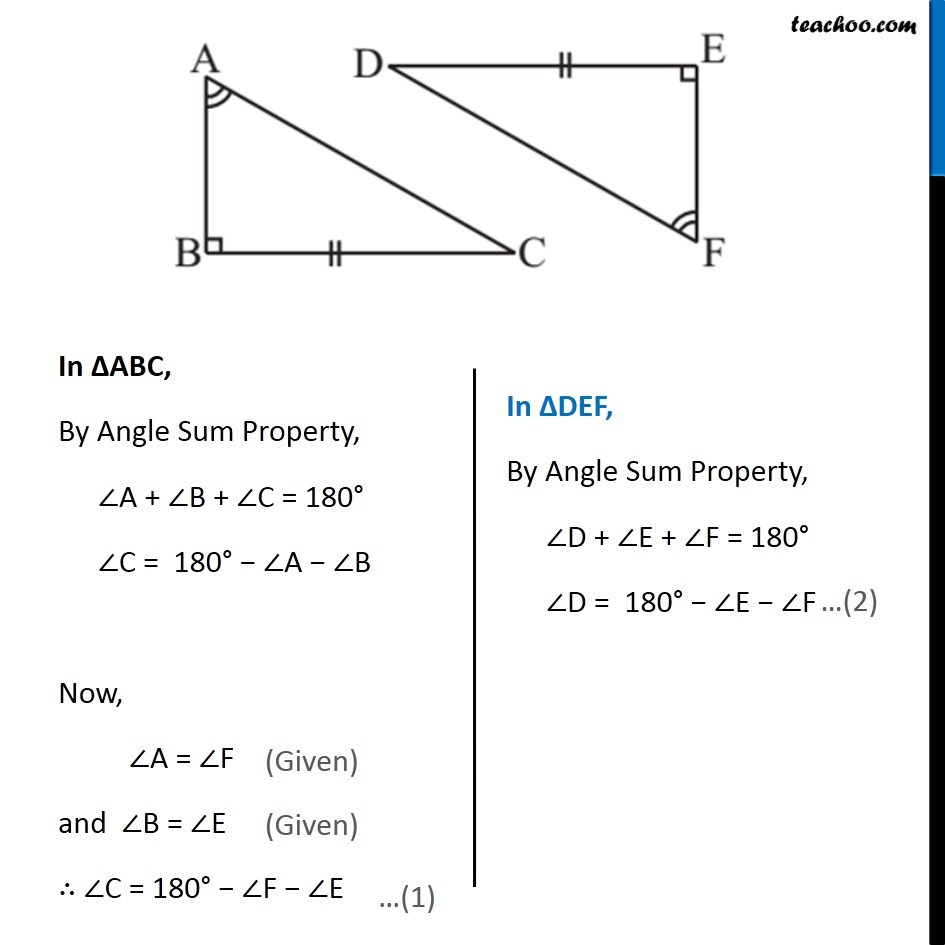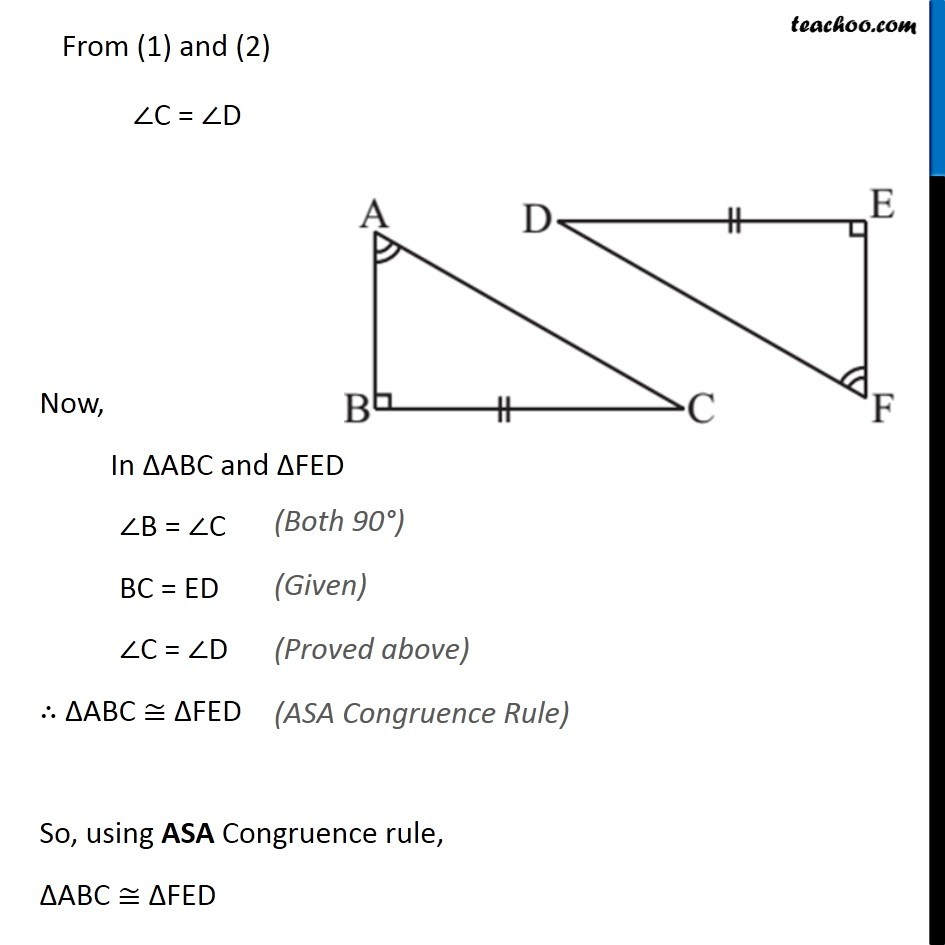Congruency Criteria Mix questions

Congruence of Triangles
Concept wiseLearn in your speed, with individual attention - Teachoo Maths 1-on-1 Class

### Transcript

Question 10 Explain, why ∆ABC ≅ ∆FED.Here, we have two angles & 1 side equal But the side is not between the angles So, finding third angle in both triangles. In ∆ABC, By Angle Sum Property, ∠A + ∠B + ∠C = 180° ∠C = 180° − ∠A − ∠B Now, ∠A = ∠F and ∠B = ∠E ∴ ∠C = 180° − ∠F − ∠E In ∆DEF, By Angle Sum Property, ∠D + ∠E + ∠F = 180° ∠D = 180° − ∠E − ∠F From (1) and (2) ∠C = ∠D Now, In ∆ABC and ∆FED ∠B = ∠C BC = ED ∠C = ∠D ∴ ∆ABC ≅ ∆FED So, using ASA Congruence rule, ∆ABC ≅ ∆FED